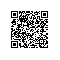# 算法中的递归分析和分治法的原理

divide（分解）：将问题划分为若干个子问题
conquer（求解）：递归地解这些子问题；若子问题Size足够小，则直接解决之
Combine（组合）：将子问题的解组合成原问题的解

①分解：把n个待排序元素划分为两个Size为n/2的子序列
②求解：递归调用归并排序将这两个子序列排序，若子序列长度为1时，已自然有序，无需做任何事情（直接求解）
③组合：将这两个已排序的子序列合并为一个有序的序列

①设完成划分的时间为D(n)
②设分解时，划分为a个子问题，每个子问题为原问题的1/b，则解各子问题的时间为aT(n/b)
③设组合时间C(n)①假定函数参数为整数，如②边界条件可忽略，当n较小时，T(n）= θ(1)

①与见过的解类似，则猜测之。
②先证较宽松的上、下界，减小猜测范围。

1、达到边界条件所需的迭代次数
2、迭代过程中的和式。若在迭代过程中已估计出解的形式，亦可用替换法
3、当递归式中包含floor和ceiling函数时，常假定参数n为一个整数次幂，以简化问题。The master method（通用法，万能法）

T(n)=aT(n/b)+f(n)   //常数a ≥1, b>1, f(n)渐近正

void up_and_down(int);//函数原型声明
int main()
{
up_and_down(1);//调用递归函数
system("pause");return 0;
}void up_and_down(int n)
{
printf("level%d, n地址=%p\n", n, &n);
if (n < 4)
{
up_and_down(n + 1);
}
printf("LEVEL%d, n地址=%p\n", n, &n);
}//计算阶乘（递归和循环）
#include <stdio.h>
#include <stdlib.h>
//计算阶乘
int factorial(int);
int loopFactorial(int);

int main()
{
int num;
printf("输入1-12的整数，q退出\n");
while (scanf_s("%d", &num))
{
if (num < 0)
{
printf("error!输入1-12的整数!");
}
else if (num > 12)
{
printf("输入1-12的整数!");
}
else
{
printf("\n%d的阶乘=%d", num, factorial(num));
printf("\n%d的阶乘=%d", num, loopFactorial(num));
}
printf("\n输入1-12的整数");
}
system("pause");
return 0;
}
//循环计算阶乘
int factorial(int n)
{
int temp;
for (temp = 1; n > 1; n--)
{
temp *= n;
}
return temp;
}
//使用递归计算阶乘
int loopFactorial(int n)
{
int temp;
if (n > 0)
{
temp = n * loopFactorial(n - 1);//属于尾递归，如n>0那么这就是最后一句
}
else
{
temp = 1;//必须要有递归结束判断条件！
}
return temp;
}

http://www.cnblogs.com/kubixuesheng/p/4355060.html使用钉钉扫一扫加入圈子
+ 订阅